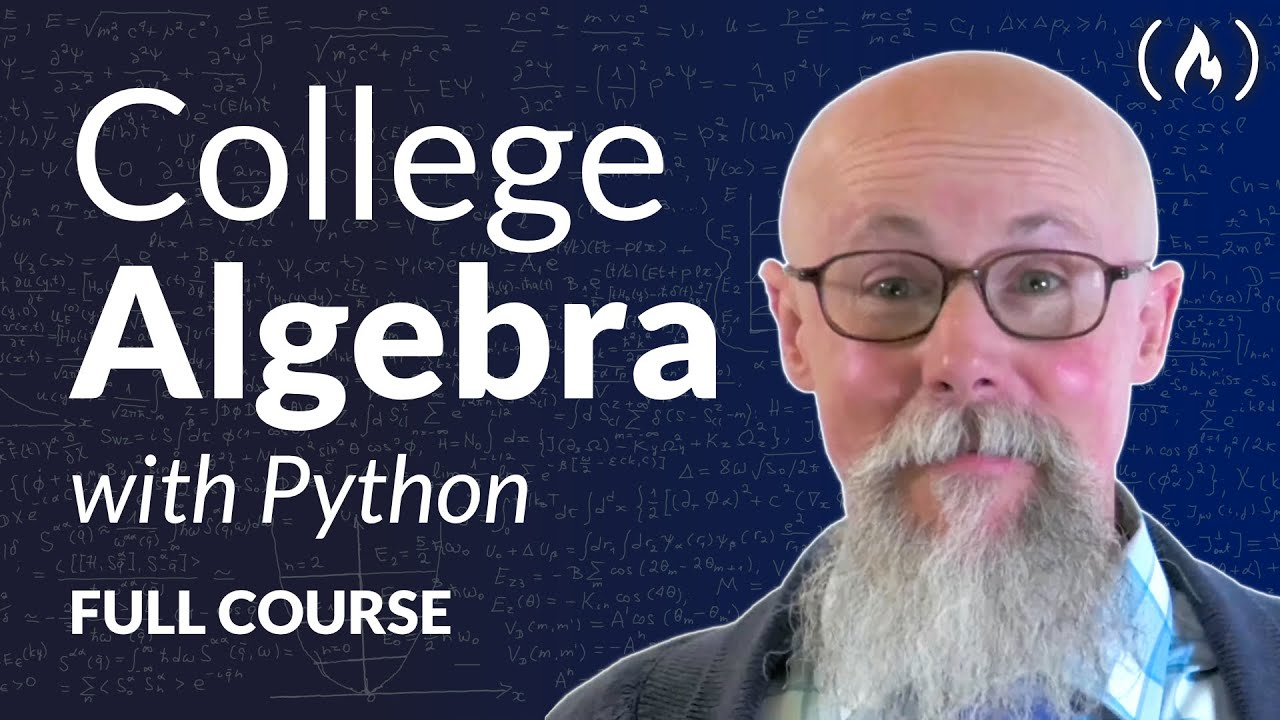# College Algebra – Full Course with Python CodeLearn college Algebra from an experienced university mathematics professor. You will also learn how to implement all the Algebra concepts using the Python programming language.

Learn college Algebra from an experienced university mathematics professor. You will also learn how to implement all the Algebra concepts using the Python programming language.

⭐️ Contents ⭐️
⌨️ (00:00:00) Introduction
⌨️ (00:14:02) Ratios, Proportions, and conversions
⌨️ (00:32:22) Basic Algebra, solving equations (one variable)
⌨️ (01:07:44) Percents, Decimals, and Fractions
⌨️ (01:40:33) Math function definition, using two variables (x,y)
⌨️ (02:17:13) Slope and intercept on a graph
⌨️ (03:28:53) Factoring, finding common factors and factoring square roots
⌨️ (05:05:40) Graphing systems of equations
⌨️ (05:36:09) Solving systems of two equations
⌨️ (06:06:17) Applications of linear systems